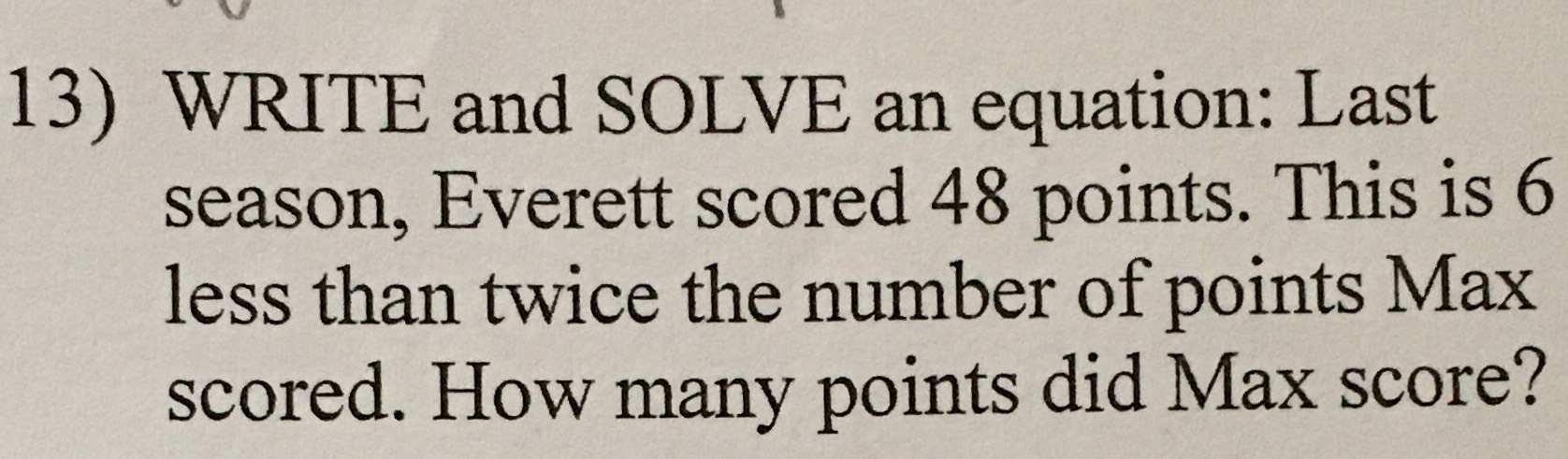### ¿Todavía tienes preguntas de matemáticas?

Pregunte a nuestros tutores expertos
Algebra
Pregunta13) WRITE and SOLVE an equation: Last season, Everett scored $$48$$ points. This is $$6$$ less than twice the number of points Max scored. How many points did Max score?